# Pythagorean theorem + square (second power, quadratic) - math problems

#### Number of problems found: 234

• Square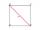Calculate the side of a square with a diagonal measurement 10 cm.
• Square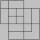Danov's father has a square of 65.25 milligram square of wire with a diagonal. How will the square be big when one mm weighs 7 mg?
• SquareCalculate area of the square with diagonal 64 cm.
• Square 2Points D[10,-8] and B[4,5] are opposed vertices of the square ABCD. Calculate area of the square ABCD.
• Diagonal of squareCalculate the side of a square when its diagonal is 10 cm.
• Square circles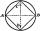Calculate the length of the described and inscribed circle to the square ABCD with a side of 5cm.
• Square s3Calculate the diagonal of the square, where its area is 0.49 cm square. And also calculate its circumference.
• SquareCalculate the perimeter and the area of square with a diagonal length 30 cm.
• Semicircle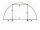To a semicircle with diameter 10 cm inscribe square. What is the length of square sides?
• Square and circlesSquare with sides 83 cm is circumscribed and inscribed with circles. Determine the radiuses of both circles.
• Square side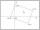Calculate length of side square ABCD with vertex A[0, 0] if diagonal BD lies on line p: -4x -5 =0.
• Circumscribed circle to squareFind the length of a circle circumscribing a square of side 10 cm. Compare it to the perimeter of this square.
• Square2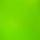Side of the square is a = 6.2 cm, how long is its diagonal?
• Annular areaThe square with side a = 1 is inscribed and circumscribed by circles. Find the annular area.
• Square diagonalCalculate the length of the square diagonal if the perimeter is 172 cm.
• Tree trunk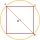What is the smallest diameter of a tree trunk that we can cut a square-section square with a side length of 20 cm?
• SquareRectangular square has side lengths 183 and 244 meters. How many meters will measure the path that leads straight diagonally from one corner to the other?
• SquarePoints A[-9,7] and B[-4,-5] are adjacent vertices of the square ABCD. Calculate the area of the square ABCD.
• FlakesA circle was described on the square, and a semicircle above each side of the square was described. This created 4 "flakes". Which is bigger: the content of the central square or the content of four chips?
• Recursion squaresIn the square, ABCD has inscribed a square so that its vertices lie at the centers of the sides of the square ABCD. The procedure of inscribing the square is repeated this way. The side length of the square ABCD is a = 22 cm. Calculate: a) the sum of peri

Do you have an interesting mathematical word problem that you can't solve it? Submit a math problem, and we can try to solve it.

We will send a solution to your e-mail address. Solved examples are also published here. Please enter the e-mail correctly and check whether you don't have a full mailbox.

Please do not submit problems from current active competitions such as Mathematical Olympiad, correspondence seminars etc...

Pythagorean theorem is the base for the right triangle calculator. Pythagorean theorem - math word problems. Square (second power, quadratic) - math word problems.